##### ACT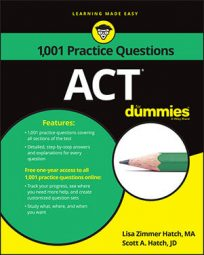The ACT Math exam requires you to have a wide range of arithmetic skills, including the ability to work with fractions. The following practice questions will test your skills at adding, dividing, and multiplying fractions, as well as converting to mixed fractions.

## Practice questions

1. What is the solution to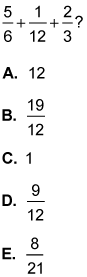2. Which of the following is equivalent to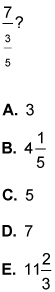1. The correct answer is Choice (B). To solve this expression, change the fractions so they have the same denominator. The least common denominator of this set of fractions is 12. The second fraction doesn’t change because its denominator is already 12.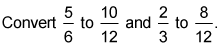Add the numerators and place the sum over the common denominator of 12: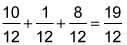2. The correct answer is Choice (E). Divide the fraction by multiplying its reciprocal: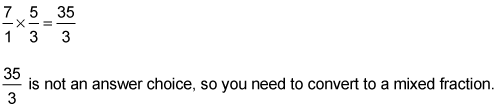Choices (A), (C), and (D) aren’t mixed fractions, so eliminate them all. When you consider the remaining two answers, you know Choice (E) must be correct even without completing the conversion. When you divide 35 by 3, you get a whole number of 11 instead of 4.